BASELINE Statement
BASELINE <OUT=SAS-data-set> <COVARIATES=SAS-data-set> <TIMELIST=list> < keyword=name ...keyword=name> </options> ;

The BASELINE statement creates a new SAS data set that contains the baseline function estimates at the event times of each stratum for every set of covariates () given in the COVARIATES= data set. If the COVARIATES= data set is not specified, a reference set of covariates consisting of the reference levels for the CLASS variables and the average values for the continuous variables is used. No BASELINE data set is created if the model contains a time-dependent variable defined by means of programming statement.

The following options are available in the BASELINE statement.

OUT=SAS-data-set

names the output BASELINE data set. If you omit the OUT= option, the data set is created and given a default name by using the DATAn convention. See the section OUT= Output Data Set in the BASELINE Statement for more information.

COVARIATES=SAS-data-set

names the SAS data set that contains the sets of explanatory variable values for which the quantities of interest are estimated. All variables in the COVARIATES= data set are copied to the OUT= data set. Thus, any variable in the COVARIATES= data set can be used to identify the covariate sets in the OUT= data set.

TIMELIST=list

specifies a list of time points at which the survival function estimates, cumulative function estimates, or MCF estimates are computed. The following specifications are equivalent:

```   timelist=5,20 to 50 by 10
timelist=5 20 30 40 50
```

If the TIMELIST= option is not specified, the default is to carry out the prediction at all event times and at time 0. This option can be used only for the Bayesian analysis.

keyword=name

specifies the statistics to be included in the OUT= data set and assigns names to the variables that contain these statistics. Specify a keyword for each desired statistic, an equal sign, and the name of the variable for the statistic. Not all keywords listed in Table 66.1 (and discussed in the text that follows) are appropriate for both the classical analysis and the Bayesian analysis; and the table summaries the choices for each analysis.

Table 66.1 Summary of the Keyword Choices

Keyword

Classical

Bayesian

Survivor Function

x

x

x

x

x

x

x

x

x

x

Cumulative Hazard Function

x

x

x

x

x

x

x

x

x

x

Cumulative Mean Function

x

x

x

x

Others

x

x

x

x

x

x

The available keywords are as follows.

CMF
MCF

specifies the cumulative mean function estimate for recurrent events data. Specifying CMF=_ALL_ is equivalent to specifying CMF=CMF, STDCMF=StdErrCMF, LOWERCMF=LowerCMF, and UPPERCMF=UpperCMF. Nelson (2002) refers to the mean function estimate as MCF (mean cumulative function).

CUMHAZ

specifies the cumulative hazard function estimate. Specifying CUMHAZ=_ALL_ is equivalent to specifying CUMHAZ=CumHaz, STDCUMHAZ=StdErrCumHaz, LOWERCUMHAZ=LowerCumHaz, and UPPERCUMHAZ=UpperCumHaz. For a Bayesian analysis, CUMHAZ=_ALL_ also includes LOWERHPDCUMHAZ= LowerHPDCumHaz and UpperHPDCUMHAZ=UpperHPDCumHaz.

LOGLOGS

specifies the log of the negative log of SURVIVAL.

LOGSURV

specifies the log of SURVIVAL.

LOWER
L

specifies the lower pointwise confidence limit for the survivor function. For a Bayesian analysis, this is the lower limit of the equal-tail credible interval for the survivor function. The confidence level is determined by the ALPHA= option.

LOWERCMF
LOWERMCF

specifies the lower pointwise confidence limit for the cumulative mean function. The confidence level is determined by the ALPHA= option.

LOWERHPD

specifies the lower limit of the HPD interval for the survivor function. The confidence level is determined by the ALPHA= option.

LOWERHPDCUMHAZ

specifies the lower limit of the HPD interval for the cumulative hazard function. The confidence level is determined by the ALPHA= option.

LOWERCUMHAZ

specifies the lower pointwise confidence limit for the cumulative hazard function. For a Bayesian analysis, this is the lower limit of the equal-tail credible interval for the cumulative hazard function. The confidence level is determined by the ALPHA= option.

STDERR

specifies the standard error of the survivor function estimator. For a Bayesian analysis, this is the standard deviation of the posterior distribution of the survivor function.

STDCMF
STDMCF

specifies the estimated standard error of the cumulative mean function estimator.

STDCUMHAZ

specifies the estimated standard error of the cumulative hazard function estimator. For a Bayesian analysis, this is the standard deviation of the posterior distribution of the cumulative hazard function.

STDXBETA

specifies the estimated standard error of the linear predictor estimator. For a Bayesian analysis, this is the standard deviation of the posterior distribution of the linear predictor.

SURVIVAL

specifies the survivor function (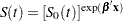) estimate. Specifying SURVIVAL=_ALL_ is equivalent to specifying SURVIVAL=Survival, STDERR=StdErrSurvival, LOWER=LowerSurvival, and UPPER=UpperSurvival; and for a Bayesian analyis, SURVIVAL=_ALL_ also specifies LOWERHPD= LowerHPDSurvival and UPPERHPD=UpperHPDSurvival.

UPPER
U

specifies the upper pointwise confidence limit for the survivor function. For a Bayesian analysis, this is the upper limit of the equal-tail credible interval for the survivor function. The confidence level is determined by the ALPHA= option.

UPPERCMF
UPPERMCF

specifies the upper pointwise confidence limit for the cumulative mean function. The confidence level is determined by the ALPHA= option.

UPPERCUMHAZ

specifies the upper pointwise confidence limit for the cumulative hazard function. For a Bayesian analysis, this is the upper limit of the equal-tail credible interval for the cumulative hazard function. The confidence level is determined by the ALPHA= option.

UPPERHPD

specifies the upper limit of the equal-tail credible interval for the survivor function. The confidence level is determined by the ALPHA= option.

UPPERHPDCUMHAZ

specifies the upper limit of the equal-tail credible interval for the cumulative hazard function. The confidence level is determined by the ALPHA= option.

XBETA

specifies the estimate of the linear predictor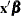.

The following options can appear in the BASELINE statement after a slash (/). The METHOD= and CLTYPE= options apply only to the estimate of the survivor function in the classical analysis. For the Bayesian analysis, the survivor function is estimated by the Breslow (1972) method.
ALPHA=value

specifies the significance level of the confidence interval for the survivor function. The value must be between 0 and 1. The default is the value of the ALPHA= option in the PROC PHREG statement, or 0.05 if that option is not specified.

CLTYPE=method
specifies the method used to compute the confidence limits for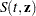, the survivor function for a subject with a fixed covariate vectorat event time. The CLTYPE= option can take the following values:
LOG

specifies that the confidence limits for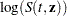be computed using the normal theory approximation. The confidence limits forare obtained by back-transforming the confidence limits for. The default is CLTYPE=LOG.

LOGLOG

specifies that the confidence limits for the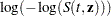be computed using normal theory approximation. The confidence limits forare obtained by back-transforming the confidence limits for.

NORMAL

specifies that the confidence limits forbe computed directly using normal theory approximation.

GROUP=variable

names a numeric variable in the COVARIATES= data set to group the baseline function curves for the observations into separate plots. This option has no effect if the PLOTS= option in the PROC PHREG statement is not specified. Curves for the covariate sets with the same value of the GROUP= variable are overlaid in the same plot.

METHOD=method
specifies the method used to compute the survivor function estimates. The two available methods are as follows:
CH
EMP

specifies that the Breslow (1972) method be used to compute the survivor function—that is, that the survivor function be estimated by exponentiating the negative empirical cumulative hazard function.

PL

specifies that the product-limit estimate of the survivor function be computed.

The default is METHOD=BRESLOW.
ROWID=variable
ID=variable
ROW=variable

names a variable in the COVARIATES= data set for identifying the baseline function curves in the plots. This option has no effect if the PLOTS= option in the PROC PHREG statement is not specified. Values of this variable are used to label the curves for the corresponding rows in the COVARIATES= data set. You can specify ROWID=_OBS_ to use the observation numbers in the COVARIATES= data set for identification.

For recurrent events data, both CMF= and CUMHAZ= statistics are the Nelson estimators, but their standard error are not the same. Confidence limits for the cumulative mean function and cumulative hazard function are based on the log transform.